# Wup 1110 10 1 Graphs of Equations SWBAT

• Slides: 8
Download presentation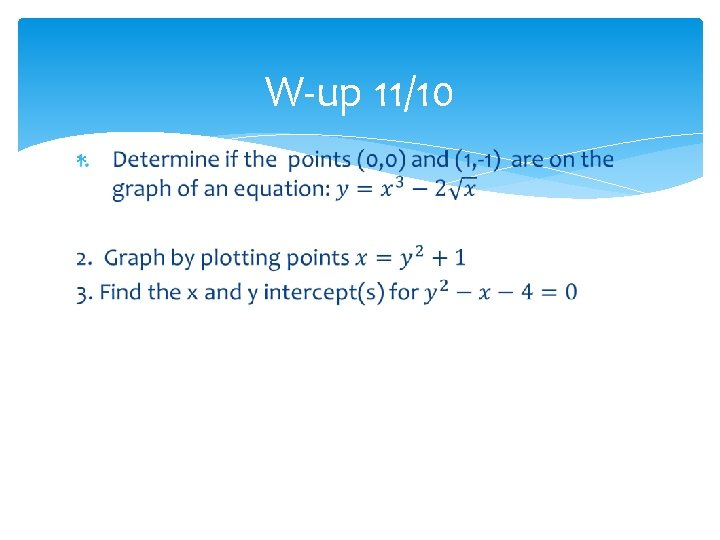W-up 11/10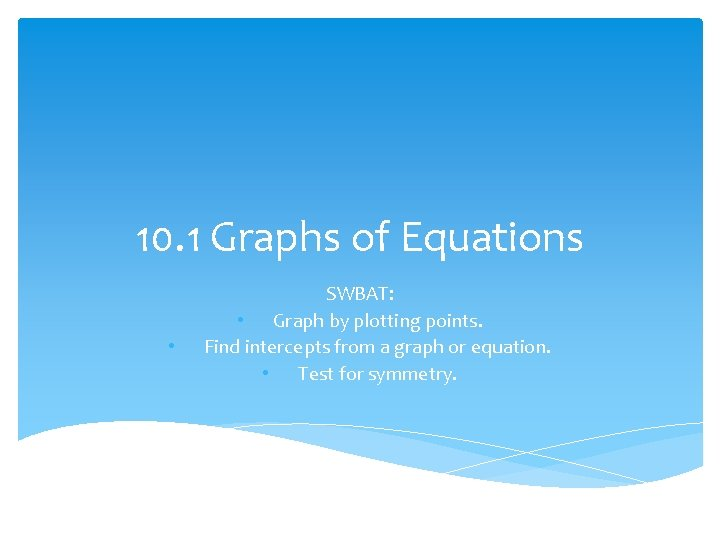10. 1 Graphs of Equations • SWBAT: • Graph by plotting points. Find intercepts from a graph or equation. • Test for symmetry.Symmetry with respect to the x-axis: For every point (x, y) on the graph, the point (x, -y) is also on the graph. To test: Replace y with –y in the equation. If it is still equivalent then it is symmetric with respect to x-axis.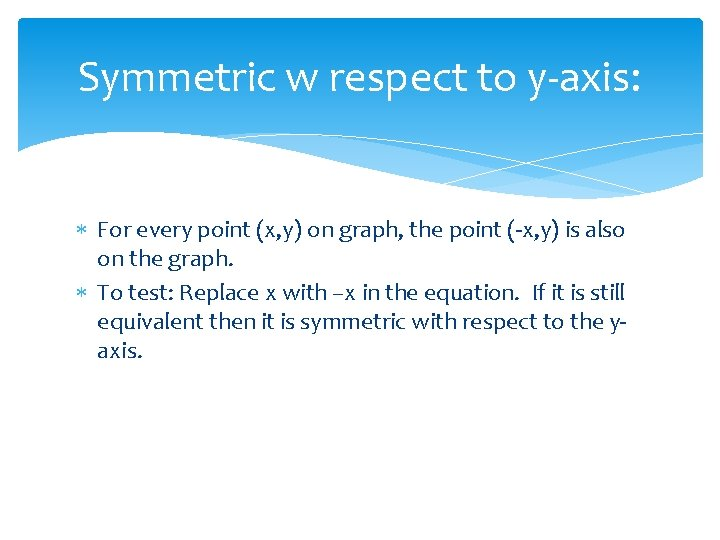Symmetric w respect to y-axis: For every point (x, y) on graph, the point (-x, y) is also on the graph. To test: Replace x with –x in the equation. If it is still equivalent then it is symmetric with respect to the yaxis.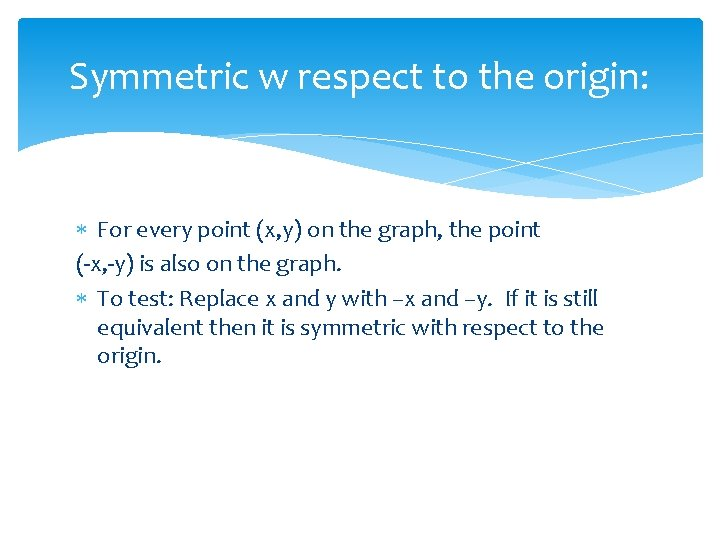Symmetric w respect to the origin: For every point (x, y) on the graph, the point (-x, -y) is also on the graph. To test: Replace x and y with –x and –y. If it is still equivalent then it is symmetric with respect to the origin.Given the graph tell if it is symmetric w respect to the x-axis, y-axis, or origin: X Y origin (3, 0) (0, 3) (-3, 0) (0, -3) Yes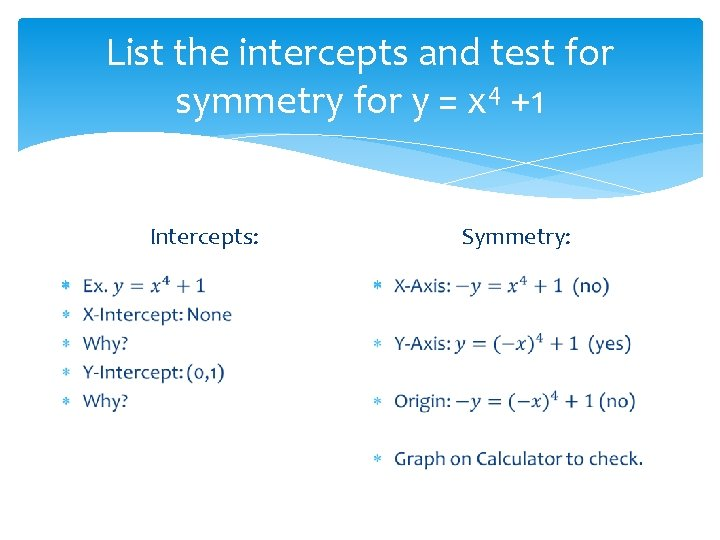List the intercepts and test for symmetry for y = x 4 +1 Symmetry: Intercepts:HW WS: 10. 1; 11 -43 odd